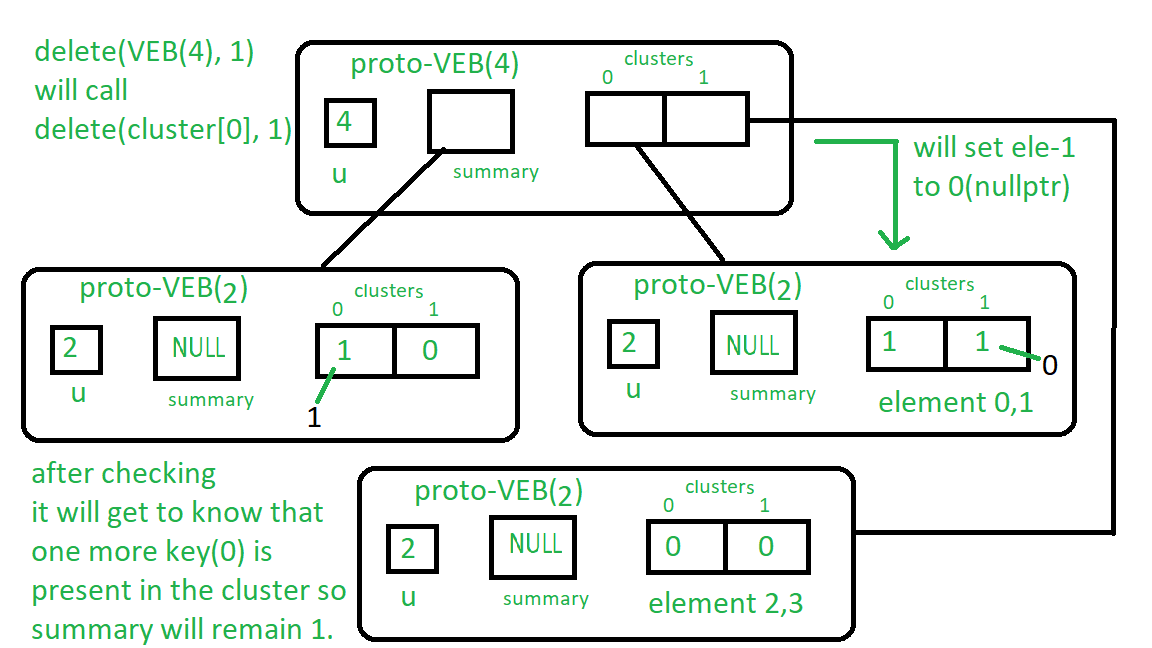Related Articles

# Proto Van Emde Boas Trees | Set 4 | Deletion

• Last Updated : 10 Sep, 2021

Please check previous sets of Proto Van Emde Boas Tree article first. It is highly recommended.
Procedure for delete:

1. Base Case: If we reach at Proto VEB with size 2 then we will check for whether the key is present or not if yes then we assign the pointer to nullptr which will set false to it presence.
2. Recursion:
• We recursively call delete function over the cluster of the keys i.e. high(key) and its position low(key).
• After we delete the key from the cluster (after we reach to the base case) we check whether there are any other keys are present in the cluster. If there is any key present then we can not set the summary to nullptr otherwise we will set the summary to nullptr by calling delete over summary.

Lets understand 1 delete on Proto-VEB of size 4:
First it will recursively call delete(cluster, 1).
So now the base case is satisfied so it will go at position 1 in the cluster Proto-VEB and will set it to nullptr if it is present.
Now we will check if any more keys are present in cluster (see the for loop in delete), 0 is present so delete(summary, 0) call is not going to execute and summary will remain same.
See the image below to understand it:
Follow the instructions written near the boxes from top to bottom.

Attention reader! Don’t stop learning now. Get hold of all the important DSA concepts with the DSA Self Paced Course at a student-friendly price and become industry ready.  To complete your preparation from learning a language to DS Algo and many more,  please refer Complete Interview Preparation Course.

In case you wish to attend live classes with experts, please refer DSA Live Classes for Working Professionals and Competitive Programming Live for Students.Below is the implementation:

## CPP

 // C++ implementation of the approach#include using namespace std; class Proto_Van_Emde_Boas {public:    // Total number of keys    int universe_size;     // Summary    Proto_Van_Emde_Boas* summary;     // Clusters array of Proto-VEB pointers    vector clusters;     int root(int u)    {        return int(sqrt(u));    }     // Function to return cluster numbers    // in which key is present    int high(int x)    {        return x / root(universe_size);    }     // Function to return position of x in cluster    int low(int x)    {        return x % root(universe_size);    }     // Function to return the index from    // cluster number and position    int generate_index(int cluster, int position)    {        return cluster * root(universe_size) + position;    }     // Constructor    Proto_Van_Emde_Boas(int size)    {        universe_size = size;         // Base case        if (size <= 2) {             // Set summary to nullptr as there is no            // more summary for size 2            summary = nullptr;             // Vector of two pointers            // nullptr in starting            clusters = vector(size, nullptr);        }        else {             // Assigning Proto-VEB(sqrt(u)) to summary            summary = new Proto_Van_Emde_Boas(root(size));             // Creating array of Proto-VEB Tree pointers of size sqrt(u)            // first all nullptrs are going to assign            clusters = vector(root(size), nullptr);             // Assigning Proto-VEB(sqrt(u)) to all its clusters            for (int i = 0; i < root(size); i++) {                clusters[i] = new Proto_Van_Emde_Boas(root(size));            }        }    }}; // Function that returns true if the// key is present in the treebool isMember(Proto_Van_Emde_Boas* helper, int key){     // If key is greater then universe_size then    // returns false    if (key >= helper->universe_size)        return false;     // If we reach at base case    // the just return whether    // pointer is nullptr then false    // else return true    if (helper->universe_size == 2) {        return helper->clusters[key];    }    else {         // Recursively go deep into the        // level of Proto-VEB tree using its        // cluster index and its position        return isMember(helper->clusters[helper->high(key)],                        helper->low(key));    }} // Function to insert a key in the treevoid insert(Proto_Van_Emde_Boas*& helper, int key){    // If we reach at base case    // then assign Proto-VEB(1) in place    // of nullptr    if (helper->universe_size == 2) {        helper->clusters[key] = new Proto_Van_Emde_Boas(1);    }    else {         // Recursively using index of cluster and its        // position in cluster        insert(helper->clusters[helper->high(key)],               helper->low(key));         // Also do the same recusion in summary VEB        insert(helper->summary, helper->high(key));    }} // Function to delete a key from the treevoid pveb_delete(Proto_Van_Emde_Boas*& helper, int key){     // Base case: If the key is present    // then make it nullptr    if (helper->universe_size == 2) {        if (helper->clusters[key]) {            delete helper->clusters[key];            helper->clusters[key] = nullptr;        }    }    else {         // Recursive delete to reach at the base case        pveb_delete(helper->clusters[helper->high(key)], helper->low(key));         bool isanyinCluster = false;         // Iterate over the cluster of keys to check whether        // any other key is present within that cluster        // If yes then we should not update summary to 0        // else update summary to 0        for (int i = helper->high(key) * helper->root(helper->universe_size);             i < (helper->high(key) + 1) * helper->root(helper->universe_size);             i++) {             // If member is present then break the loop            if (isMember(helper->clusters[helper->high(key)], i)) {                isanyinCluster = true;                break;            }        }         // If no member is present then        // update summary to zero        if (isanyinCluster == false) {            pveb_delete(helper->summary, helper->high(key));        }    }} // Driver codeint main(){    Proto_Van_Emde_Boas* hello = new Proto_Van_Emde_Boas(4);     cout << isMember(hello, 2);     insert(hello, 2);     insert(hello, 3);     cout << isMember(hello, 2);     pveb_delete(hello, 2);     cout << isMember(hello, 2);}

Recurrence Relation for Delete:

T(u) = T(u) = 2T()) + O(log2())

Time Complexity : O(log2(u)*log2(log2(u)))

My Personal Notes arrow_drop_up